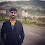### New Year Chaos Solution - The Coding Shala

Home >> Programming Questions >> New Year Chaos

## New Year Chaos Problem Solution

In this post, you will learn how to solve New Year Chaos Problem and implement its solution in Java.

Problem: It's New Year's Day and everyone's in line for the Wonderland rollercoaster ride! There are a number of people queued up, and each person wears a sticker indicating their initial position in the queue. Initial positions increment from at the front of the line to at the back.
Any person in the queue can bribe the person directly in front of them to swap positions. If two people swap positions, they still wear the same sticker denoting their original places in line. One person can bribe at most two others. For Example, if n=8 and Person 5 bribes Person 4, the queue will look like this: 1,2,3,5,4,6,7,8.
Fascinated by this chaotic queue, you decide you must know the minimum number of bribes that took place to get the queue into its current state.

Input:
2
5
2 1 5 3 4
5
2 5 1 3 4
Output:
3
Too chaotic

## New Year Chaos Solution in Java

In this problem, we need to check two things first is need to check if a person is moved from their original position or not. if a person is moved from their original position but not more than two positions because only a maximum of two bribes is allowed then we calculate the number of bribes.

#### Approach / Explanation

First, eliminate the chaotic case. Any person who is more than 2 positions ahead[left side] from their original position can not be there so return "Too chaotic" for that.

First, let's try to understand what we need to find here. If a person is not standing at their original position that means either the person has bribed someone and jump ahead[left side] or every person with a number greater than me who is now standing ahead of me[left side] has bribed me at some point.

we know only a maximum of two bribes allowed so a person can only move back by number-2. For num, inner loop will be [num-2, arr.length-1] or [0, arr.length-1] (if num-2<0). The bribe count is all the numbers that are greater than the current number.

Let's understand this by an example:

current position: {1,2,5,3,7,8,6,4}
initial position:   {1,2,3,4,5,6,7,8}

Here, person 4 is not its original position so all the numbers that are greater than 4 must have bribed 4 at some time and move to the left. 4 have [6,8,7, and 5] numbers ahead that mean these people must have bribed 4 and moved to left.

Note: Here 4 has not bribed anyone because it is not ahead of its original position.

Like this 7, and 8 are ahead of 6 and we can find for others in the same way.

In the above example the total number of bribes are:

for 4: 4 (6,8,7,5 are ahead).
for 6: 2 (8,7 are ahead).
for 8: 0
for 7: 0
for 3: 1 (5 is ahead)
for 5: 0
for 2: 0
for 1: 0
so the total number of bribes is 7.

So we need to check through the queue in front of you and count all the numbers that are greater than you and that are the total number of bribes.

Java Program:

```import java.io.*;
import java.math.*;
import java.security.*;
import java.text.*;
import java.util.*;
import java.util.concurrent.*;
import java.util.regex.*;

public class Solution {

// Complete the minimumBribes function below.
static void minimumBribes(int[] q) {
int ans = 0;
for(int i=q.length-1;i>=0;i--){
int ch_pos = q[i]-(i+1);
if(ch_pos>2) { System.out.println("Too chaotic");
return; }
else{```
`                //find starting index`
```                //range[num-2, arr.length] or 0 to length
int st = Math.max(0,q[i]-2);
for(int j=st;j<i;j++){
if(q[j]>q[i]) ans++;
}
}
}
System.out.println(ans);
}

private static final Scanner scanner = new Scanner(System.in);

public static void main(String[] args) {
int t = scanner.nextInt();
scanner.skip("(\r\n|[\n\r\u2028\u2029\u0085])?");

for (int tItr = 0; tItr < t; tItr++) {
int n = scanner.nextInt();
scanner.skip("(\r\n|[\n\r\u2028\u2029\u0085])?");

int[] q = new int[n];

String[] qItems = scanner.nextLine().split(" ");
scanner.skip("(\r\n|[\n\r\u2028\u2029\u0085])?");

for (int i = 0; i < n; i++) {
int qItem = Integer.parseInt(qItems[i]);
q[i] = qItem;
}

minimumBribes(q);
}

scanner.close();
}
}
```

Other Posts You May Like

1.it will be great if you explain solution

2.int st = Math.max(0,q[i]-2);
for(int j=st;jq[i]) ans++;
}
can you please explain logic behind this code?

1.Approach and the logic behind this code added.